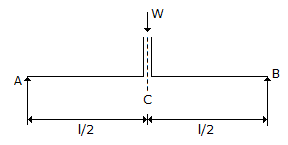# Civil Engineering - UPSC Civil Service Exam Questions

41.

According to Boussinesq's theory, the vertical stress at a point in a semi-infinite soil mass depends upon

 A. point load, coordinates of the point and modulus of elasticity of soil B. point load, coordinates of the point, modulus of elasticity of soil and its Poisson's ratio C. point load and coordinates of the point D. point load, coordinates of the point, modulus of elasticity of soil and its density.

Explanation:

No answer description available for this question. Let us discuss.

42.

A star is observed at its western elongation. If the latitude of place of observation is θ, the declination of star is δ, altitude of star at elongation is α, then the hour angle of star is given by

 A. cos H = cos δ . sec θ B. cos H = tan θ . cot δ C. cos H = tan θ . cot α D. cos H = tan δ . cot θ

Explanation:

No answer description available for this question. Let us discuss.

43.

For the simply supported beam in the figure, C is the centre of the span. C is also the point through which the resultant of the column load W passes. The column rests on the beam over a small length symmetrically on either side of C. What is the shearing force at C ?A. W/2 B. W/4 C. W D. 0

Explanation:

No answer description available for this question. Let us discuss.

44.

A 20 m chain was found to be 10 cm too long after chaining a distance of 2000 m. It was found to be 18 cm too long at the end of the day's work after chaining a total distance of 4000 m. What is the true distance if the chain was correct before the commencement of the day's work ?

 A. 3962 m B. 4019 m C. 3981 m D. 4038 m

Explanation:

No answer description available for this question. Let us discuss.

45.

Timber can be made reasonably fire-resistant by

 A. soaking it in Ammonium Sulphate B. coating with Tar paint C. pumping creosote oil into timber under high pressure D. seasoning process